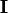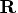Previous Page | Next Page

 Language Reference

## Index Creation Operator:   :

creates an index vector

value1:value2

The index creation operator (:) creates a row vector with a first element that is value1. The second element is value1+1, and so on, as long as the elements are less than or equal to value2. For example, the following statement produces the vector, as shown:

```
I=7:10;
```

```
I             1 row       4 cols    (numeric)

7         8         9        10
```
If value1 is greater than value2, a reverse-order index is created. For example, the following statement produces the vector, as shown:

```
r=10:6;
```

```
R             1 row       5 cols    (numeric)

10          9         8         7        6
```
The index creation operator also works on character arguments with a numeric suffix. For example, consider the following statement:
```
varlist='var1':'var5';
```
This statement produces the following result:
```
VARLIST    1 row       5 cols    (character, size 4)

var1  var2  var3  var4  var5
```
Use the DO function if you want an increment other than 1 or -1.Previous Page | Next Page | Top of Page